# 14-1100/Homework Assignment 3

Jump to: navigation, search

This assignment is due at class time on Thursday, November 6, 2011.

### Solve the following questions

Problem 1. (easy)

1. Prove that in any ring,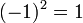$(-1)^2=1$.
2. Prove that even in a ring without a unit,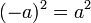$(-a)^2=a^2$.

(Feel free to do the second part first and then to substitute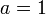$a=1$).

Problem 2.

1. (Qualifying exam, April 2009) Prove that a finite integral domain is a field.
2. (Qualifying exam, September 2008) Prove that in a finite commutative ring, every prime ideal is maximal.

Problem 3. (Dummit and Foote) A ring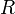$R$ is called a Boolean ring if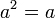$a^2=a$ for all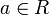$a\in R$.

1. Prove that every Boolean ring is commutative.
2. Prove that the only Boolean ring that is also an integral domain is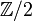${\mathbb Z}/2$.

Problem 4. (Selick) In a ring$R$, an element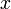$x$ is called "nilpotent" if for some positive$n$,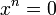$x^n=0$. Let$\eta(R)$ be the set of all nilpotent elements of$R$.

1. Prove that if$R$ is commutative then$\eta(R)$ is an ideal.
2. Give an example of a non-commutative ring$R$ in which$\eta(R)$ is not an ideal.

Problem 5. (comprehensive exam, 2009) Let$A$ be a commutative ring. Show that a polynomial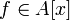$f\in A[x]$ is invertible in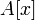$A[x]$ iff its constant term is invertible in$A$ and the rest of its coefficients are nilpotent.

Problem 6. (bonus) Let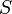$S$ be the ring of bounded sequences of real numbers with pointwise addition and multiplication, let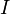$I$ be the ideal made of all sequences that are equal to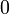$0$ except in at most finitely many places, and let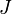$J$ be a maximal ideal in$S$ containing$I$.

1. Prove that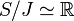$S/J\simeq{\mathbb R}$.
2. Denote by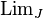$\operatorname{Lim}_J$ the projection of$S$ to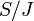$S/J$ composed with the identification of the latter with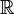${\mathbb R}$, so that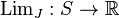$\operatorname{Lim}_J:S\to{\mathbb R}$. Prove that for any scalar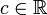$c\in{\mathbb R}$ and any bounded sequences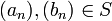$(a_n),(b_n)\in S$, we have that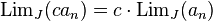$\operatorname{Lim}_J (ca_n)=c\cdot\operatorname{Lim}_J(a_n)$,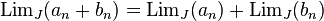$\operatorname{Lim}_J(a_n+b_n)=\operatorname{Lim}_J(a_n)+\operatorname{Lim}_J(b_n)$, and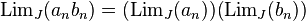$\operatorname{Lim}_J(a_nb_n)=(\operatorname{Lim}_J(a_n))(\operatorname{Lim}_J(b_n))$. (Easy, no bonuses for this part).
3. Prove that if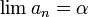$\lim a_n=\alpha$ in the ordinary sense of limits of sequences, then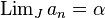$\operatorname{Lim}_Ja_n=\alpha$.
4. Is there a$J$ for which$\operatorname{Lim}_J$ is also translation invariant, namely such that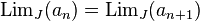$\operatorname{Lim}_J(a_n)=\operatorname{Lim}_J(a_{n+1})$? (Again, easy).

Warning. The right order for solving these questions is not necessarily the order in which they are presented.

Opinion. The sum total of all that is that using the axiom of choice you can construct things that are both too good to be true and not really useful anyway. Blame the axiom of choice, don't blame me.

### Term Test

The MAT 1100 Core Algebra I Term Test took place on Monday October 20 at 1:10PM at Bahen 6183. Here's the PDF: TT-1100.pdf.

The average grade is about 77.5/100 and the standard deviation is about 20. The complete list of grades is as follows:

100 100 100 99 98 96 94 89 76 70 65 63 61 60 56 54 37

### Appeals

Remember! Grading is a difficult process and mistakes always happen - solutions get misread, parts are forgotten, grades are not added up correctly. You must read your exam and make sure that you understand how it was graded. If you disagree with anything, don't hesitate to complain!

The deadline to start the appeal process is Thursday October 30 at 2:30PM.

 Dror's notes above / Student's notes below